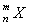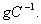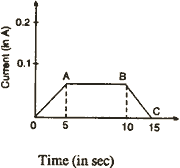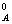Monday 26th October 2020
 CBSE Guess > Papers > Question Papers > Class XII > 2003 > Physics > Delhi Set -II PHYSICS—2003 (Set II—Delhi)
Note: Except for the following questions, all the remaining questions have been asked in Set I. Q. 1. A wire of resistivity 'p' is stretched to twice its length. What will be its new resistivity? Q. 2. Define one Henry. Q. 3. Two heater wires of the same dimensions are first connected in series and then in parallel to a source of supply. What will he the ratio of heat produced in the two cases? Q. 4. How does the (i) pole strength and (ii) magnetic moment of each part of a bar magnet change if it is cut into two equal pieces along its length? Q. 7. A nucleusemits one alpha particle and one beta particle. Find the mass number and atomic number of the product nucleus. Q. 11. What is an electric line of force? Sketch lines of force due to two equal positive charges placed at a small distance apart in air. Q. 12. In a copper voltameter, a varying electric current, as shown in graph, is passed. The mass of copper deposited at the end of 30 seconds is m grams. Using the graph, find the value of e.c.e. of copper inQ. 13. How do we make the choice of a communication channel? A message signal has a bandwidth of 5 MHz. Suggest a possible communication channel for its transmission. Q. 17. Why is interference pattern not detected, when two coherent sources are far apart? In Young's experiment, the width of the fringes obtained with light of wavelength 6000is 2.0 mm. Calculate the fringe width if the entire apparatus is immersed in a liquid medium of refractive index 1.33. Q. 25. Draw a circuit diagram of a common emitter amplifier using p-n-p transistor. Show graphically input and output voltages separately. The current gain for common emitter amplifier is 69. If the emitter current is 7.0 mA, find (i) base current and (ii) collector current. Physics 2003 Question Papers Class XII Delhi Outside Delhi Compartment Delhi Compartment Delhi Set I Set I Set I Set I Set II Set II Set II Set II CBSE 2003 Question Papers Class XII